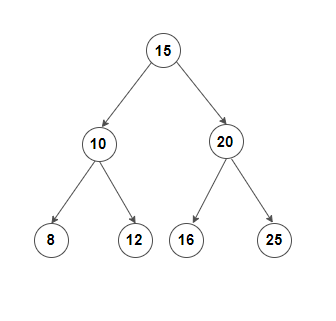1

# Predecessor And Successor In BST

Difficulty: EASYContributed By
Souhard Swami
Avg. time to solve
10 min
Success Rate
90%

Problem Statement

#### You have been given a binary search tree of integers with ‘N’ nodes. You are also given 'KEY' which represents data of a node of this tree. Your task is to find the predecessor and successor of the given node in the BST.

##### Note:
``````1. The predecessor of a node in BST is that node that will be visited just before the given node in the inorder traversal of the tree. If the given node is visited first in the inorder traversal, then its predecessor is NULL.

2. The successor of a node in BST is that node that will be visited immediately after the given node in the inorder traversal of the tree. If the given node is visited last in the inorder traversal, then its successor is NULL.

3. The node for which predecessor and successor are to be found will always be present in the given tree.

4. A binary search tree (BST) is a binary tree data structure which has the following properties.
• The left subtree of a node contains only nodes with data less than the node’s data.
• The right subtree of a node contains only nodes with data greater than the node’s data.
• Both the left and right subtrees must also be binary search trees.
``````
##### Input Format:
``````The first line contains an integer 'T' which denotes the number of test cases or queries to be run. Then the test cases follow.

The first line of each test case contains the elements of the tree in the level order form separated by a single space. If any node does not have a left or right child, take -1 in its place. Refer to the example for further clarification.

The second line of each test case contains 'KEY' representing the data of the node for which predecessor and successor are to be found.
``````
##### Example:
``````Elements are in the level order form. The input consists of values of nodes separated by a single space in a single line. In case a node is null, we take -1 in its place.

For example, the input for the tree depicted in the below image would be :
````````````1
2 3
4 -1 5 6
-1 7 -1 -1 -1 -1
-1 -1

Explanation :
Level 1 :
The root node of the tree is 1

Level 2 :
Left child of 1 = 2
Right child of 1 = 3

Level 3 :
Left child of 2 = 4
Right child of 2 = null (-1)
Left child of 3 = 5
Right child of 3 = 6

Level 4 :
Left child of 4 = null (-1)
Right child of 4 = 7
Left child of 5 = null (-1)
Right child of 5 = null (-1)
Left child of 6 = null (-1)
Right child of 6 = null (-1)

Level 5 :
Left child of 7 = null (-1)
Right child of 7 = null (-1)

The first not-null node (of the previous level) is treated as the parent of the first two nodes of the current level.

The second not-null node (of the previous level) is treated as the parent node for the next two nodes of the current level and so on.

The input ends when all nodes at the last level are null (-1).
``````
##### Note :
``````The above format was just to provide clarity on how the input is formed for a given tree.

The sequence will be put together in a single line separated by a single space. Hence, for the above-depicted tree, the input will be given as:

1 2 3 4 -1 5 6 -1 7 -1 -1 -1 -1 -1 -1
``````
##### Output Format :
``````For each test case, print two single space-separated integers representing data values of the predecessor and the successor node, respectively. If any of the two doesn’t exist, print -1 in place of it.

Print output of each test case in a separate line.
``````
##### Note:
``````You are not required to print anything explicitly. It has already been taken care of. Just implement the function.
``````
##### Constraint :
``````1 <= T <= 100
1 <= N <= 5000
0 <= data <= 10^6 and data != -1

Time Limit: 1 sec
``````
##### Sample Input 1:
``````1
15 10 20 8 12 16 25 -1 -1 -1 -1 -1 -1 -1 -1
10
``````
##### Sample output 1:
``````8 12
``````
##### Explanation of Sample output 1:
``````The tree can be represented as follows:
````````````The inorder traversal of this tree will be 8 10 12 15 16 20 25.

Since the node with data 8 is on the immediate left of the node with data 10 in the inorder traversal, the node with data 8 is the predecessor.

Since the node with data 12 is on the immediate right of the node with data 10 in the inorder traversal, the node with data 12 is the successor.
``````
##### Sample Input 2:
``````2
10 5 -1 -1 -1 -1
5
20 -1 -1
20
``````
##### Sample output 2:
``````-1 10
-1 -1
``````Console